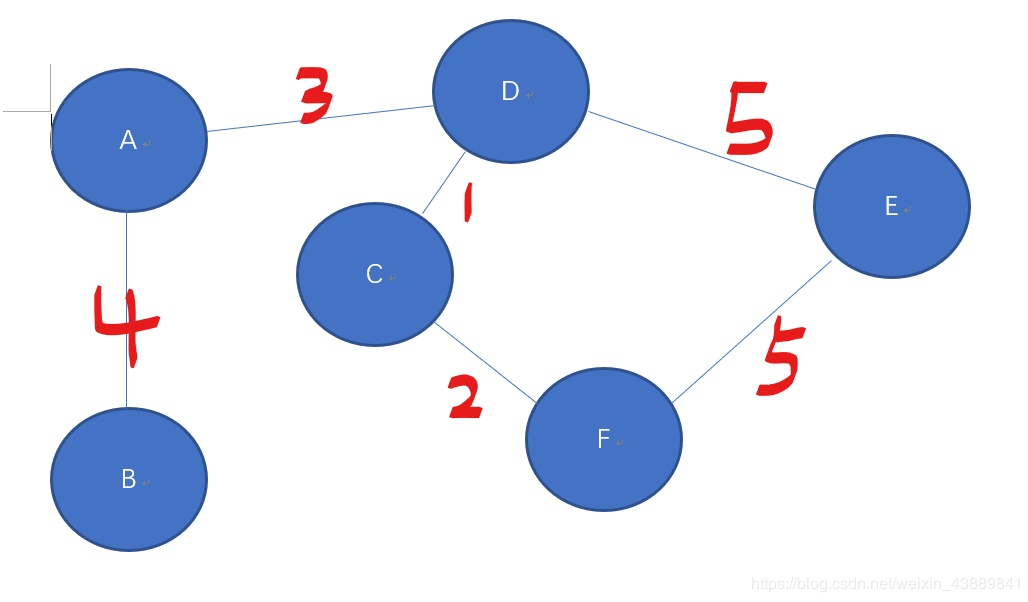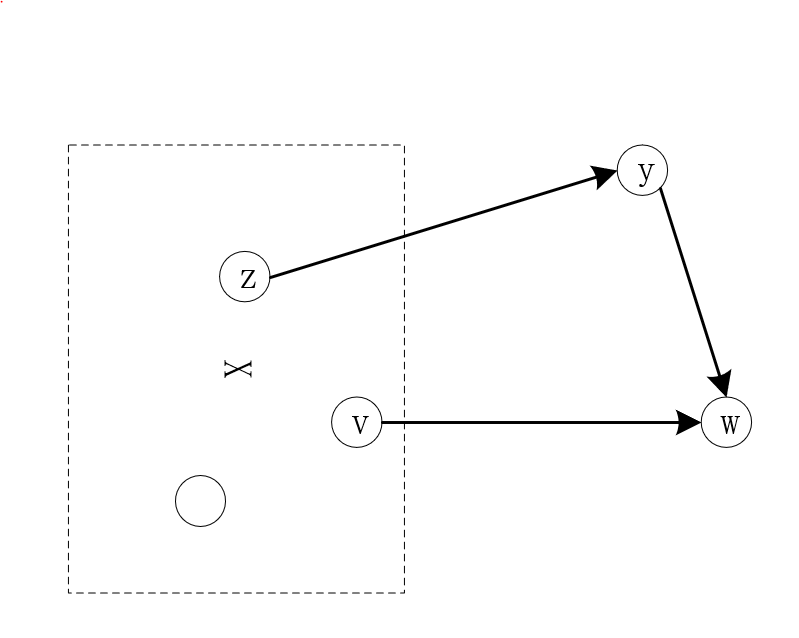• #include&lt;stdio.h&gt; #include&lt;stdlib.h&gt; #define max 11000000000 inta; intd;//d表示某特定边距离 intp;//p表示永久边距离 ...scan...
#include<stdio.h>

#include<stdlib.h>

#define max 11000000000

inta;

intd;//d表示某特定边距离

intp;//p表示永久边距离

inti,j,k;

intm;//m代表边数

intn;//n代表点数

intmain()

{

scanf("%d%d",&n,&m);

intmin1;

intx,y,z;

for(i=1;i<=m;i++)

{

scanf("%d%d%d",&x,&y,&z);

a[x][y]=z;

a[y][x]=z;

}

for(i=1;i<=n;i++)

d[i]=max1;

d=0;

for(i=1;i<=n;i++)

{

min1=max1;

for(j=1;j<=n;j++)

if(!p[j]&&d[j]<min1)

{

min1=d[j];

k=j;

}

p[k]=j;

for(j=1;j<=n;j++)

if(a[k][j]!=0&&!p[j]&&d[j]>d[k]+a[k][j])

d[j]=d[k]+a[k][j];

}

for(i=1;i<n;i++)

printf("%d->",p[i]);

printf("%d\n",p[n]);

return0;

}

展开全文acm
• Dijkstra(迪杰特斯拉） 离散数学学过，不会就去跟着书上模拟一遍就完事儿（深度上进行操作） 使用条件 不能出现负权值路径 用邻接矩阵实现 #include <bits/stdc++.h> using namespace std; const int maxn=...
Dijkstra(迪杰特斯拉）
离散数学学过，不会就去跟着书上模拟一遍就完事儿（深度上进行操作）

使用条件

不能出现负权值路径
用邻接矩阵实现
#include <bits/stdc++.h>

using namespace std;
const int maxn=105,inf=1e9+7;
int mp[maxn][maxn];//图的邻接矩阵
int dis[maxn];//记录移动
bool vis[maxn];//标记
int n,m;//点、边

void DJ(int s){
for(int i=1;i<=n;i++)
dis[i]=inf;

memset(vis,0,sizeof(vis));
dis[s]=0;
vis[s]=1;

int turn=n;
while(turn--){
//Findmin
int target=-1,minDis=inf;
for(int i=1;i<=n;i++)
if(!vis[i] && dis[i]<minDis)//找到所以可以走的边的最小权值
{
target = i;
minDis = dis[i];
}
//合并
vis[target] = 1;//找到最小后标记

//Update
for(int i=1;i<=n;i++){
if(mp[target][i]!=inf && !vis[i])//如果存在边且没有被访问过
dis[i] = min(dis[i],dis[target]+mp[target][i]);//该点的权值和前继点所有的权值取最小值
}
}
}

int main()
{
cin>>n>>m;
//初始化图
for(int i=1;i<=n;i++)
for(int j=1;j<=n;j++)
if(i!=j)
mp[i][j]=mp[j][i]=inf;//相等部分，全局变量已自动初始化为0
//存图
for(int i=1;i<=m;i++){
int u,v,w;
cin>>u>>v>>w;
mp[u][v]=mp[v][u]=w;//无向图
//mp[u][v]=w;有向图
}

DJ(1);
cout<<dis[n]<<endl;
return 0;
}

用邻接表实现(链式前向星)
#include <bits/stdc++.h>

using namespace std;

const int maxn=200005,inf=2147483647;
long long dis[maxn];
int vis[maxn];
int cnt=0;
int n,m,s;//点、边

struct edge{
int v;
int next;
int weight;
}edge[maxn];

edge[cnt].v = v;
edge[cnt].weight = w;
}

void DJ(int s){
for(int i=1;i<=n;i++)
{
dis[i]=2147483647;
}

memset(vis,0,sizeof(vis));
dis[s]=0;

int turn=n;
while(turn--){
//Findmin
int target=s,minDis=inf;
for(int i=1;i<=n;i++)
if(!vis[i] && dis[i]<minDis)//找到所以可以走的边的最小权值
{
target = i;
minDis = dis[i];
}
//合并
vis[target] = 1;//找到最小后标记

//Update
if(!vis[edge[i].v])//如果没有被访问过
if(dis[edge[i].v]>dis[target]+edge[i].weight)
dis[edge[i].v] = dis[target]+edge[i].weight;//该点的权值和前继点所有的权值取最小值
}
}
}

int main()
{
cin>>n>>m>>s;
//初始化图
for(int i=1;i<=m;i++){
int u,v,w;
cin>>u>>v>>w;
}
DJ(s);
for(int i=1;i<=n;i++)
cout<<dis[i]<<" ";
return 0;
}

堆优化（用优先队列实现）
关于优先队列
priority_queue<int> q;//从大到小
priority_queue<int, vector<int>, greater<int>>q;//从小到大
//自定义优先级
struct edge{
int v;
int weight;
bool operator < (const edge A) const{//不能漏掉第二个const
return weight > A.weight;
}
};

priority_queue<edge> Q;//默认大根堆，需要重载成小根堆

关于Vector嵌套及遍历
vector<vector<int> > v;//v的每个元素都是一个整形动态数组

//for + iterator
vector<int> v1 = {0,1,2,3,4,5,6};

for(vector<int>::iterator x = v1.begin(); x != v1.end(); x++)
{
cout << *x <<" ";
}
//for + auto
vector<int> v1 = {0,1,2,3,4,5,6};

for(auto x	: v1)
{
cout << x <<" ";
}
//for + 下标
vector<int> v1 = {0,1,2,3,4,5,6};

for(int i = 0; i < v1.size(); i++)
{
cout << v1[i] <<" ";
}

优化
#include <bits/stdc++.h>

using namespace std;

const int maxn=200005,inf=1e9+7;
int dis[maxn];
bool vis[maxn];
int n,m,s;//点、边
struct edge{
int v;
int weight; //在优先队列中是dis[v]的值；如果表示边，那么就是边权
bool operator<(const node A) const
//不能漏掉第二个const，会导致底层错误
/*
template<typename _Tp>
struct less : public binary_function<_Tp, _Tp, bool>
{
_GLIBCXX14_CONSTEXPR
bool
operator()(const _Tp& __x, const _Tp& __y) const(这行是底层模板)
{ return __x < __y; }
};
*/
{
return weight > A.weight;
}
};

priority_queue<node> Q;//默认大根堆，需要重载成小根堆
vector<vector<node> > V;

void DJ(int s)
{
//fill(dis, dis + n + 1, inf);
for(int i=1;i<=n;i++)
{
dis[i]=inf;
}
dis[s] = 0;
memset(vis, 0, sizeof(vis));
Q.push({s, dis[s]});//v，dis[v]
while (!Q.empty())
{
//FindMin
node now = Q.top();
Q.pop();
if (vis[now.v])//如果访问过，就换下一个点
continue;
//合并
vis[now.v] = 1;//找到最小后标记

//Update
for(auto x : V[now.v]){
if(!vis[x.v] && dis[x.v] > dis[now.v]+x.weight){
dis[x.v] = dis[now.v]+x.weight;
Q.push({x.v,dis[x.v]});
}
}
}
}

int main()
{
cin>>n>>m>>s;

//初始化图
V.resize(n+1);
for (int i = 1; i <= n; i++)
V[i].clear();
for(int i=1;i<=m;i++){
int u,v,w;
cin>>u>>v>>w;
V[u].push_back({v,w});
}

DJ(s);
for(int i=1;i<=n;i++)
cout<<dis[i]<<" ";
return 0;
}

展开全文dijkstra
• 迪杰特斯拉算法：求源点到任何一个其他点的最短路径 弗洛依德算法：可以看成是缔结特斯拉算法的一个扩展版，对迪杰特斯拉算法进行for循环就是佛洛依德算法。 首先来说大概思路： 上图是一个无向图，假设A是你在的...
迪杰特斯拉算法：
求源点到任何一个其他点的最短路径
思想：红字代表两点之间的距离。
上图是一个无向图，假设A是你在的地方，也就是源点，你想知道你到其他的地方的最短路径，设置ABCDEF编号分别对应为123456。
设定一个数组flag来表示每个点是否被访问过，初始化都为0，表明都没被访问过  设置一个存储A到各点距离的数组D[N]，如果无法两点之间达，则设置为正无穷大。例如对于上图来说，AF两点距离为正无穷，AB则为一个整数  设置一个存储前驱的数组P[N]（方便存储路径顺序，就是可以得出来两点之间最短路径具体是怎么走的）  如果源点到某一点”有路“，例如A点到B点有路，那么可以设置P中对应B的数组值为A点的编号。 第一步： 找到距离我们当前位置A点最近的点，且该点未被访问。对于上图，最近的点是D。 第二步： 以刚找到的点作为中介点，进行距离的更新。 对于上图，本来AC不可达，也就是无穷大，然后我们对A->C和A->D->C进行比较，发现后者是4，所以更新AC为4。 C也可以到达F，对A-F和A->D->C->F进行比较，发现A->F是无穷大，所以更新A->F对应的D是6. 循环上面两步： C没有可达的点了，继续找到距离我们当前位置A点最近的点，且该点未被访问，找到B，再继续对B可达的点进行判断，更新D[N]
弗洛依德算法：
给定图G，求任意两点之间的距离
思路：假设两个点A和B，找一个中间点C，如果A和B之间的直接距离小于AC+CB，那就说明绕一下反倒更近，更新
C++实现：
#include <iostream>
#include <bits/stdc++.h>
#define Max 1000
#define inf 100000;
using namespace std;
/* run this program using the console pauser or add your own getch, system("pause") or input loop */

int G[Max][Max];
int main(int argc, char** argv) {

int n,m;//定义点的数量和边的数量
cin>>n>>m;
int begin,end,value;
//初始话都是不可达
for(int i=0;i<n;i++)
{
for(int j=0;j<n;j++)
{
G[i][j]=inf;
}
}
for(int i=0;i<m;i++)
{
cin>>begin>>end>>value;
G[begin][end]=value;
G[end][begin]=value;

}
for(int k=0;k<n;k++)
for(int i=0;i<n;i++)
for(int j=0;j<n;j++)
{
if(G[i][j]>G[i][k]+G[k][j])
{
G[i][j]=G[i][k]+G[k][j];
}
}
//打印输出
for(int i=0;i<n;i++)
{
for(int j=0;j<n;j++)
{
cout<<G[i][j]<<" ";
}
cout<<endl;
}
return 0;
}

结论
对迪杰特斯拉算法进行for循环也可以获取任意一点到任意一点的最短路径无论是迪杰特斯拉算法还是弗洛依德算法，若要实现相同的求任意两点之间最短路径，时间复杂度都是O(n三次方)
展开全文数据结构
• 上一文中我们谈到了单源路径算法 迪杰特斯拉算法。 今天我们就来继续讲一下这个算法的正确性。如果有点忘记的同学们可以先点回去复习一下，尤其是注意一下迪杰特斯拉算法的贪婪规则。 我们怎么证明该算法的正确性...
上一文中我们谈到了单源路径算法 迪杰特斯拉算法。
今天我们就来继续讲一下这个算法的正确性。如果有点忘记的同学们可以先点回去复习一下，尤其是注意一下迪杰特斯拉算法的贪婪规则。
我们怎么证明该算法的正确性呢？老规矩，我们使用数学归纳法(一般想不到什么好办法，就用这个办法吧)。
当X为空时，我们会选择与起点s点最近的点加入X，显然s到该点的最近路径选择是正确的。
我们假设已经有一个X了，且所有X中节点对应的最短路径以及长度均正确存储在A[v], B[v]中。
现在我们假设新加入一个点w时， 存在一条额外的路径使得该路径比迪杰特斯拉算法选择的路径还要更短。如上图所示，我们假设v到w是算法选择的路径。注意此时y点不在X中。大家还记得迪杰特斯拉算法选择新的点加入X中的原则吗？我当时还强调了一下，上文中用红字显示的。即在所有从X出发的边中选择使得 最小的(v, w)。 大家有没有发现我们是选择的一条边啊，表面上看起来是选择点加入X这个集合中。但是从X出发的边是不是有很多条，且起点可以是v 也可以是Z啊，因为v和z都在X中。
那么这里，如果算法选择了v到w，那么也就意味着v到w的距离小于，显然就更加小于
也就说明v到w 才是到w的最短路径。与我们前面的假设就矛盾了。至此我们证明了该算法的正确性。


展开全文• 发现迪杰特斯拉的优化过一段时间不写就直接忘光光了，写个博客加深一下印象（这里默认是双向模板） 基础的迪杰特斯拉就不写了，只写优化 首先是这部分 void add(int x,int y,int z){ to[++count1]=y; v[count1]=z;...算法
• 迪杰特斯拉 #include<iostream> #include<queue> using namespace std; const int maxn = 1e5+10; const int INF = 1e9; struct edge{ int from,to,value; edge(int a,int b,int c){ from =a; to=...算法 队列 dijkstra
•   迪杰特斯拉还是比较常用的最短路径算法。之前学数据结构时写的代码很冗余，总是记不住。今天有时间，整理出一个简练的模板吧！   实现的方式对应了优先队列，模板中包括建图的方法（其实哪种都可以）。本文用...
• 前言：通过JavaScript实现该算法，并求出初始点到各个点的最短路径<!DOCTYPE html> 迪杰特斯拉算法 //说明 //@xiaoD //计算第javascript 算法 dijkstra算法
• 无向图 最短路径：两顶点之间经历的边数最少的路径 网图 最短路径：两顶点之间经历的边上的权值之和最短的路径 迪杰特斯拉算法思路：
• 在C语言的实训中，我学习到了一个自己以前曾经想学但是碍于水平不够未学习的算法：迪杰特斯拉算法。通俗地说，就是解决最短路径问题。相信大家都有过这样的经历：从a城市到b城市有一段距离，从a城市到c城市也有一段...
• 迪杰特斯拉算法每一步需要选取离X最近的边添加入X集合中。而求解这个最小值如果能够很高效，那么可以进一步提高算法的效率。 由于我们每一步选取最小的，因此我们可以计算出剩余节点离X中点的距离，然后用最小堆的...
• 迪杰特斯拉算法对比弗洛伊德算法 转载于:https://www.cnblogs.com/LoveFishC/archive/2013/06/14/3846895.html
• 数据结构之C++实现最短路径迪杰特斯拉(dijkstra)算法.md #include <iostream> #include <cstdlib> #include <cmath> #include <ctime> #include "sys/io.h" using namespace std; #define ...数据结构 算法 c++ dijkstra
• 用C语言实现最短路径迪杰特斯拉算法（Dijkstra） 1、参考文章 https://blog.csdn.net/u014535666/article/details/82870854 2、路径图： 3、 （1）m[i][j]图 （2）dis[I]数组（部分）：表示目前点1到点i的最短距离...c语言
• #include<stdio.h> #include<stdlib.h> int i, j, n, m, max = 10000, sum, x, y, z, k; int a, d, p; int main() { scanf("%d%d", &n, &m); for (i = 1;...}shell
• #include #include #include #include #include"stdio.h" #include #include #include using namespace std; ...#define INFINITY 32767 ...//迪杰特斯拉算法的辅助数组 int have ; //存放景点信息 string i
• 今天写了一道可以用迪杰特斯拉算法实现的题目，题目如下： 给你n个点，m条无向边，每条边都有长度d和花费p，给你起点s终点t，要求输出起点到终点的最短距离及其花费，如果最短距离有多条路线，则输出花费最少的，...
• ## 迪杰特斯拉算法

千次阅读 2017-08-06 18:40:32
Dijkstra(迪杰斯特拉)算法是典型的单源最短路径算法，这个算法我主动学了三遍，第一主动学的时候，是看严蔚敏的《数据结构》，当时应该是学懂了，有点透彻地理解了这个算法，但是没有记录下来，后来就忘记了， ...
• 求最短路径长度-迪杰特斯拉算法   Dijkstra(迪杰斯特拉)算法是典型的最短路径路由算法，用于计算一个节点到其他所有节点的最短路径。主要特点是以起始点为中心向外层层扩展，直到扩展到终点为止。 Dijkstra算法能...算法 数据结构
• Dijteska算法 求1-->n的最小距离 辅助数据 N : 所有点集合 S : 已计算距离的结合 M[i][j]: i-->j的距离值,-1表示不可达 D[]:距离数组 D =0,D[i] = +inf,{i!... for i in (N-S) ...
• 回头我研究了两三个小时的迪杰特斯拉算法，终于研究出了一套规律，彻彻底底搞懂了我迪哥博大精深的思维，下面我就详细讲解一下。  首先附上代码和注释   int dis ;//start到i的最短距离 int a
• 最短路径---Dijkstra迪杰特斯拉算法---《数据结构》严蔚敏最短路径 Dijkstra 数据结构严蔚敏
• 迪杰特斯拉算法堆优化 #include using namespace std; /*Dijkstra算法： *1.初始化dis[start]=0,其他点,dis[]=无穷大;所有点初始化le[]=0 *2.在le[]=0的里面找dis最小的点x,令le[x]=1; *3.更新x的邻接点y,(x,y)边的...dijkstra 数据结构...